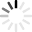# Perimeter Quizzes & Trivia

A perimeter is a path that surrounds a two-dimensional shape. The term may be used either for the path or its length - it can be thought of as the length of the outline of a shape. So, now that you remember what it’s about, let’s move on to math and test your geometry knowledge. A bit nervous, are you? You shouldn’t be, unless you skipped those classes back in the day.

Do you remember the perimeter formulas for all shapes? How do you call the perimeter of a circular or an ellipse? What does a wetter perimeter means? Do you remember the answers? Do you think you’re ready for perimeter quizzes? Take our quizzes and have fun with the questions!
Top Trending

Here are a couple of practice questions that I would like you to do! Good Luck! Brandi

Questions: 5  |  Attempts: 2423   |  Last updated: Jan 19, 2021
• Sample Question
If we have a triangle with the side lengths of 3, 4, 5, find the perimeter.In the mathematical context, the perimeter is the line that surrounds a plane figure with two dimensions. Put, it is the measure of the space a shape will occupy. The perimeter of a circular figure is its circumference. What is...

Questions: 10  |  Attempts: 398   |  Last updated: May 27, 2019
• Sample Question
What mathematical operation is exclusively performed in obtaining the perimeter of a triangle?Quiz Description

Questions: 5  |  Attempts: 176   |  Last updated: May 19, 2014Related